# Examples of Concrete, Semi-concrete, iconic, or pictorial, and Formal operational, abstract, or symbolic Representations in mathematical proofs (proof of odd + odd = even)

This page includes three examples of an algebraic proof for the addition of two odd numbers resulting in an even sum. Examples include:

## Concrete Representation of Odd + Odd = Even sum

Problem or conjecture

When any two odd numbers are added, it will result in an even sum.

Young learners will operationalize and demonstrate this with concrete objects.

Background information

Learners can

• count and represent groups of objects to 20.
• know even and odd numbers to 20.
• know an even number of objects is put into pairs without any left over.
• know odd numbers of objects is put into pairs with one left over

Problem

• What kind of number do you get when you add two odd numbers? even
• What kind of number is the sum of two odd numbers? even
• Is that always true? yes
• How can you convince others it is? show them

Solution

Example of how young learners use counters or other objects to solve:

Clan you show me what kind of number you get when you add two odd numbers?

I'll use counters.

Grabs a handful...Creates two groups.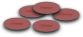Counts out a group of seven and a group of five.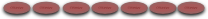How can you convince me they both are odd numbers?

I'll put them in pairs and show there is a left over.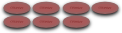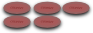Okay. Continue.

I'll add them ... (push them together)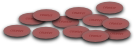and see if they are even or odd (pair them to see if there are or are not any extras.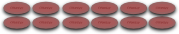There are six pairs of twelve counters with no extra.

If you tell them they showed that 7 + 5 is even and ask.

Do you think it is the same when you add any two odd numbers?

Different learners will answer differently. Most will say they need to try it and want to try another. Others may be confused and not know. And a very small few might say yes.

If you probe, they may say. The pairing will be the same (pairs plus one) for all odd numbers and if you have two odd, then the two extra will make a pair. Most young will not generalize this until older, when they have more experience with numbers and knowledge to operate with larger chunks of information and use a less concrete representations. Such as the following example. Iconic or semi-concrete representations to solve.

## Semi-concrete or Iconic (pictorial or illustration) Representation of Odd + Odd = Even sum

Problem or conjecture

When any two odd numbers are added, it will result in an even sum.

Background information

Learners

• count and represent groups of objects to 100.
• know even and odd numbers to 100.
• know an even number of objects is put into pairs without any left over.
• know odd numbers of objects is put into pairs with one left over.
• know a proof is an explanation of how or why.

Problem

• What kind of number do you get when you add two odd numbers? even
• What kind of number is the sum of two odd numbers? even
• Is that always true? yes
• How can you convince others it is? draw a picture to show them

Solution

Example of how intermediate learners use drawings and larger chunks of information to solve:

Can you think of a rule or conjecture you can prove for adding two odd numbers?

Well say for example you add. 7 + 5

I'll draw seven lines in one group for seven and five lines in another group.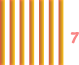+I can show they are odd by pairing them.Seven is odd, because there are six pairs and an extra.

Five is odd, because there are two pairs and an extra.

When I add them there will be the same pairs and the two extra will make another pair.Which makes six groups and no extra.Therefore, odd + odd = even

Will it work with all different odd numbers?

Yes. Whenever two odd groups are paired, each group will have one left over which can be put together to make another pair.

So if you add any two odd valued numbers or groups of objects, each will always have a remaining single, from each group, to put together to make another pair so it will be even.

Good. What general statements can you make about all the different ways odd and even numbers can be added and their sums?

• If even numbers are added, they are all paired to begin with and can stay paired. Because adding two even numbers will result in pairs with no extras when they are put together. Result: even + even = even
• If there is an even number and an odd number are added, one group will be paired and the other will have an extra. When they are put together, the extra will still not be paired. Result: even + odd = odd
• Odd we did. If odd numbers are added, the two not paired numbers can be paired. Result odd + odd = even

## Formal operational or symbolic or abstract Representation of Odd + Odd = Even sum

Problem or conjecture

When any two odd numbers are added, it will result in an even sum.

Background information

Learners

• count and represent groups of objects to 100.
• know even and odd numbers to 100.
• know an even number of objects is put into pairs without any left over.
• know odd numbers of objects is put into pairs with one left over.
• know a proof is an explanation of how or why.
• know proofs can be generalized for all numbers of a set if the are defined that way.

Problem

• What kind of number do you get when you add two odd numbers? even
• What kind of number is the sum of two odd numbers? even
• Is that always true? yes
• How can you write a proof that will convince others it is? use equations to show the sum of two odd numbers is always an even number.

Solution with symbols

Example of how upper middle level and high school (formal operational) learners can use symbols and larger chunks of information to solve:

Write a mathematical proof for the conjecture:

Adding any two odd numbers (addends) always results in an even sum.

Learners initial response is often similar to ...

I'll use x and y (symbols) to represent two different odd numbers.

• X can represent one odd number
• Y can represent the other odd number

If both are odd, and odd numbers are: 1, 3, 5, 7, 9, 11, 13, …

Then X and Y would be among them so

X + Y = Z

Z would be even: 0, 2, 4, 6, 8, 10, ...

Teacher's reflection on learner development

Learners who haven't had a lot of experience with making equations for different numbers will be stumped as to how to represent any odd number with an equation. Experiences for learners on how to describe patterns of numbers can be found in even odd patterns & other pattern teaching notes and activities.

For learners who have had experiences writing equations or statements for patterns may still need a little prompt, try something like:

Notice each number is two more than the previous number. If you use n to represent a starting number can you think of something to do with it so that any number inserted for n will result in an odd number?

Which should lead to the idea of n + 2.

However, that will and won’t work … because …

• If n is an odd number, then it will work.
• If n isn’t an odd number, then it won’t work. For example, if an even number is somehow included, for whatever reason, then it would become even not odd (that’s odd).
• N + 2 for 0, 1, 2, 3, 4, … would be
0+2=2; 1+2=3; 2+2=4; 3+2=5; 4+2=6; …

How can the even be removed?

To understand how adding two or multiplying by two is different, learners must understand multiplication and division as unitizing. A concept beyond the idea of multiplication as repeated addition constructed with many experiences of generating multiples, factors, and the relationships between them. Experiences which will enable learners to systematically generate reasonable possibilities of multiplicative equations for real world situation.

If learners are successful and get n + 2, then they can plug it into the X + Y equation to see if it works.

I hear wondering:

Basically what is needed is an equation that will leave holes or skip numbers when we substitute numbers (0, 1, 2, 3, 4, ...) .

Since the counting numbers increase by one, addition won't work so if we use multiplication that will skip numbers.

The hole size needed, is 2, so maybe multiply by 2.

Oh (n * 2) will give even numbers. (0, 2, 4, 6, 8, 10, ...)

Oh! Oh! odd numbers have one left over so what if we add one?

Say what?

Like: (N * 2) + 1

Okay.

Let’s make a function table for it and see …

n * 2 + 1 = X or Y

• 0 * 2 + 1 = 1
• 1 * 2 + 1 = 3
• 2 * 2 + 1 = 5
• 3 * 2 + 1 = 7
• 4 * 2 + 1 = 9
• 5 * 2 + 1 = 11
• 6 * 2 + 1 = 13
• 7 * 2 + 1 = 15

When learners get to here. They have all the pattern needed to create and understand a proof. All they need is to use the equation and insert it into their conjecture or original equation or formula.

Let's use this equation, (n * 2) + 1, for the odd numbers in our original equation or conjecture:

Xodd + Yodd = even number?

Use the equation (n * 2) + 1 for the two odd numbers: X & Y.

((X * 2) + 1) + ((Y * 2) + 1) = even?

Simplify by multiplying 2 time X and 2 times Y and adding the ones.

2X + 2Y + 2 =

Notice all the TWOS!

We know from earlier n * 2 will always be even for any n number so 2X and 2Y are always even and 2 is even so 2X + 2Y + 2 is an even number.

And. if we factor 2, we get ...

2*(X + Y + 1)

And any number with a factor of two is even and all even numbers have a factor of two as we know from the Fundamental Theorem of Arithmetic.

Which proves adding odd numbers sums to an even number.

Cool :-)

Of course this is a perfect dialog and life in a classroom or with one or more learners isn't perfect, but it provides a possible map for a way to prove odd plus odd results in even.

Is the following example of odd and even concrete, semi concrete, or symbolic? Support your answer.

 ODD EVEN OD D EV EN